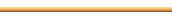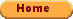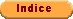Ten lessons I wish I had learned before I started teaching differential equations. Invited address delivered at the meeting of the Mathematical Association of America, Simmons College, Thursday, April 24 1997. by Gian-Carlo Rota Professor of Applied Mathematics and Philosophy Mathematics Department, MIT

One of many mistakes of my youth was writing a textbook in ordinary differential equations. It set me back several years in my career in mathematics. However, it had a redeeming feature: it led me to realize that I had no idea what a differential equation is. The more I teach differential equations, the less I understand the mystery of differential equations.
One of several unpleasant consequences of writing such a textbook is my being called upon to teach the sophomore differential equations course at MIT. This course is justly viewed as the most unpleasant undergraduate course in mathematics, by both teachers and students. Some of my colleagues have publicly announced that they would rather resign from MIT than lecture in sophomore differential equations. No such threat is available to me, since I am incorrectly labeled as the one memher of the department who is supposed to have some expertise in the subject, guilty of writing an elementary textbook still in print.
The Administative Director of the MIT mathematics department who exercises supreme authority upon the faculty 's teaching has only to wave a copy of my book at me, while staring at me in silence. At her prompting, I bow and fall into line; I will be the lecturer in the dreaded course for one more year, and I will repeat the mistakes I have been making every year since I first taught differential equations in 1958.
It is hopeless to expect that I will correct any of my mistakes at this stage of life. To allay my feelings of guilt, I will resort to a ruse. I will present them to you in the attractive literary form of the decalogue. The goofs, gaffes misunderstandings and prejudices I am about to list are not exactly hot off the press, and you may find them cloyingly familiar. Why, then, make a public spectacle of them?. Well I myself always find it gratifying to listen to opinions I agree with, and I surmise that you may feel likewise as you listen to my tirade.
Lesson one: Most of the material now taught in an introductory differential equations course is hopelessly obsolete.
Some time ago, I received a review copy of Cauchy's introductory course in differential equations, reprinted by Springer on the anniversary of Cauchy's death. Cauchy taught his course in the middle of the nineteenth century, and his lecture notes were written in the attractive, flowing style in which mathematicians of his time used to write.
It was a pleasure to read familar topics written up by one of the great mathematicians of the past century. But it was also a surprise to discover how little the content of the course has changed since Cauchy. Practically the only change has been the introduction of systems, which have made their way down the ladder since my days as a graduate student.
As I read Cauchy's textbook I realized how much of the material we now teach is obsolete. The order of presentation of the outworn topics has not been altered. The most preposterous items are found at the beginning when the text (any text) will list a number of disconnected tricks that are passed off as useful, such as exact equations, integrating factors, homogeneous differential equations, and similarly preposterous techniques". Since it is rare - to put it gently - to find a differential equation of this kind ever occurring in engineering practice, the exercises provided along with these topics are of limited scope: as a matter of fact the same sets of exercises have been coming down the pike with little change since Euler. Lecturers in the course most of whom are unaware of any applications of differential equations beyond those given in elementary texts, scrupolously follow the traditional order of the material, as if it were a religious rite; their ignorance of the broader theory of ordinary differential equations makes them sensitive to change.
Why is it that no one has undertaken the task of cleaning the Augean stables of elementary differential equations? I will hazard an answer: for the same reason why we see so little change anywhere today, whether in society in politics, or in science. Vested interests dominate every nook and cranny of our society, even the society of mathematicians. A revamped elementary differential equations course would require Professor Neanderthal at Oshkosh College to learn the subject anew. The fatuous, expensive, multi-colored texthooks that are now cornering the market would be forced out of print. New textbooks would have to be written. We know what an effort goes into writing a textbook, and how negatively such an effort is rewarded. No clear-headed young mathematician will risk ruining his or her career by writing such a book, as I did.
The sophomore course in differential equations will never be reformed. It will die of natural death, and it will be replaced by several shorter courses that will deal with realistic aspects of differential equations. It is to be hoped that these new courses will be taught by mathematicians rather than by engineers: the budget of any mathematics department is entirely dependent on the number of engineering students enrolled in our elementary courses. Were it not for these courses, which engineers generously defer to mathematicians, our mathematics departments would be doomed to extinction.
Lesson two: reduce to a minimum the discussion of first-order differential equations at the beginning of the course.
One of my favored mathematics books is Boole's "Differential Equations", publisbed at about the same time as Cauchy's, and reprinted by that great benefactor of mathematics, the Chelsea Publishing Company. About half the book is devoted to the solution of the first order differential equations, with dazzling pyrotechnics that no one has matched since.
None of Boole's beautiful techniques is of any conceivable use to anyone who deals with differential equations today. Only two of them have survived: separation of variables and changes of variables. Integrating factors have become a joke, although engineers occasionally put on a show in defense of them (more about this later).
Never in my life have I heard of anyone solving a first order differential equation by finding an integrating factor. Despite such negative evidence, we spend one or more lectures on integrating factors, while telling the students with a straight face that they are important.
Lesson three: Linear differential equations with constant coefficients are the bottom line.
This lesson is subdivided into two lessons: first, make sure that the students learn bow to solve linear differential equations with constant coefficients. This is a basic item of mathematical literacy. Even the worst students must learn to solve a linear differential equation of the second order with constant coefficients. It is one of the teacher's inescapable duties.
Second. linear differential equations with variable coefficients should be weeded out. Why? For the following four reasons:
1. With the exception of the Euler-Cauchy differential equation, namely, the differential equation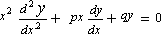no other second order linear differential equation of the second order can be solved explicitly, unless one introduces special functions. Some thirty or so years ago, Bessel functions were included in the syllabus, but in our day they are out of the question.
Teaching a subject of which no honest examples can be given is, in my opinion, demoralizing.
2. One of the most beautiful chapters of mathematics is the Sturm-Liouville theory of second order differential equations. Theorems on separation of zeros, minimax properties, existence of eigenvalues and eigenfunctions were once thought to have great educational value, and were included in every treatment of differential equations, no matter how elementary.
One day, two realizations came to me as a shock. First, I realized that the beautiful theorems of Sturm and Liouville are of no use whatsoever. To be sure, these theorems have been a great source of inspiration to research mathematicians: the theory of totally positive matrices grew out of them, and Chebyshev systems are now practically a chapter in combinatorics. Morse theory is a chapter of topology that grew out of Sturm-Liouville theory.
3. A worse realization was in store. As we teach second order linear differential equations with variable coefficients, we have in the hack of our minds eigenvalues and eigenfunctions. The spectral theory of non singular Sturm-Liouville systems on a finite interval can be presented by fairly elementary methods, including a proof of completeleness of eigenfunctions. Such presentations are stilì to be found in courses bearing titles like "Mathematical methods in engineering" (and, I must shamefully admit, in my own book).
I can assure you that there is not one instance of a non-singular Sturm-Liouville eigenvalue problem on a finite interval that occurs anywhere in mathematics, physics or engineering. All Sturm-Liouville systems that occur in mathematics, physics, or engineering are singular, and a presentation of their theory that pretends to a minimum of rigor requires notions of spectral theory that are beyond not only the first but the second course in differential equations.
To conclude: everything we have always taught about second order linear differential equations with non constant coefficients is utterly devoid of relevance.
4. Should we then let the students remain blissfully unaware of the existence of linear differential equations with non constant coefficients? If not, is there anything we can say about such differential equations at the elementary level?
From time to time I succumb to one of the untapped temptations of the theory of differential equations: differential algebra. No elementary presentation of this beautiful subject has ever been attempted to the best of my knowledge; Cohen's books of the twenties is the closest, and it is still eagerly (and secretly) read today.
Let me stick my neck out and propose that two results of differential algebra might be appreciated even by students in an elementary course. I will state one by way of end of this already long lesson, and reserve the second one for the next lesson.
I have always felt excited when telling the students that even though there is no formula for the general solution of a second order linear differential equation, there is nevertheless an explicit formula for the Wronskian of two solutions. The Wronskian allows to find a second solution if one solution is known (by the way, this is a point on which you will find several beautiful examples in Boole's text). But there is a more fundamental fact, which I will state in a mathematical form that needs to be bowdlerized if we ever decide to try it out on an elementary class. It states that every differential polynomial in the two solutions of a second order linear differential equation which is independent of the choice of a basis of solutions equals a polynomial in the Wronskian and in the coefficients of the differential equation (this is the differential equations analog of the fundamental theorem on symmetric functions, but keep it quiet).
Lesson four: Teach changes of variables.
Whatever else the students will need in later life, it is certain that they will have to handle changes of variables for both first order and second order differential equations. One should spend some time teaching in wealth of detail relevant changes of variables. Luckily, some of these are still included in texthooks, though no textbook now in print awards this essential technique the importance it deserves. Worse, no one realizes that changes of variables are not just a trick; they are a coherent theory (it is the differential analog of classical invariant theory, but let it pass).
For second order linear differential equations, formulas for changes of dependent and independent variables are known, but such formulas are not to be found in any book written in this century, even though they are of the utmost usefulness.
Liouville discovered a differential polynomial in the coefficients of a second order linear differential equation which he called the invariant He proved that two linear second order differential equations can be transformed into each other by changes of variables if and only if they have the same invariant This theorem is not to be found in any text. It was stated as an exercise in the first edition of my book, but my coauthor insisted that it be omitted from later editions.
Lesson five: Forget about existence and uniqueness of solutions.
Allow me to state another controversial opinion: existence theorems for the solutions of ordinary differential equations are not as important as they are cracked up to be. They are "psychological theorems" instances of those results of mathematics that make little difference, but which satisfy our psychological cravings for something to grab. As a matter of fact, the need for proving existence theorems was not felt until the end of the nineteenth century, and I refuse to believe that someone like Cauchy or Riemaun did not think of them. More probably, they thought about the possibility of proving existence theorems, but they rejected it as inferior mathematics.
Existence theorems would be far more interesting if there existed examples of ordinary differential equations which do not have solutions. (This happens for partial differential equations, where existence theorems are extraordinarily interesting.
Uniqueness theorems are a touchier point. I feel guilty when I have to state to the students without proof that every solution of a second order linear differential equation with constant coefficients is a linear combination of two solutions.
Once in a while I present in class the proof of the fact that all solutions of the differential equation
y' = ay

are of the form y = ceax, but I have never succeeded in making the proof convincing. Most often, some student will retort with the dreaded question: "So what?". I have resisted the temptation to give the matrix analog of this result, which would prove uniqueness for systems, and hence for all linear differential equations with constant coefficients. I don't see any way out of this impasse. Lesson six: Linear systems with constant coefficients are the meat and potatoes of the course.
Solving linear systems with constant coefficients is the most important technique the students learn in a differential equations course. No matter what field of study a student will choose in science or technology they are bound to run into large linear Systems. The computerization of the solution of large systems makes it all the more important that the students should be aware of the theory, including eigenvalues and eigenvectors of matrices, exponentials of matrices, and whatever goes with that.
Here again we meet with a lack of relevant examples. A lot of interesting systems with constant coefficients have been discovered in the last thirty years, in control, in economics, in signal processing, even in mathematics. None of these attractive examples is presently included in introductory texts. At present all examples of matrix systems one finds in such texts are either planar or else they are artificial.
There are ritualistic items in the chapter on systems that should be ruthlessly weeded out. The much-trumpeted method of variation of parameters is pathetically useless. It is hard even to assign problems the students can work out. Let the students learn it properly if they ever learn Feynman diagrams.
The older version of the method of variation of parameters that 'people pretended to use in solving inhomogenous second order linear differential equations with variable coefficients is perhaps the worst scandal in the history of ordinary differential equations. It has been copied for centuries word for word from one textbook to the next until the present day with the same artificial examples (there are no other examples, by the way). This pathetic argument was pawned off to thousands of unsuspecting classes before the fundamental role of Green's functions was recognized it is still to be found in several textbooks and Professor Neanderthal loves it.
Lesson seven: Stay away from differentials.
I come now to my "bète noire": integrating factors. The way integrating factors are presented in textbooks since 1800 is nothing short of scandalous. We have the means to give a rigorous, enlightening presentation of the method that does not require any handwaving and does not appeal to yet-to-be-defined "differential forms". I will take unfair advantage ot the time you have granted me to describe the full extend of the dishonesty involved in the old presentations, and to sketch the elementary argument that should replace them.
The preposterous description of integrating factors goes as follows. In order to solve the first order differential equation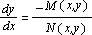rewrite the differential equation in "differential form" (whatever that means)

Mdx + Ndy = 0.

We justify this sudden introduction of differentials by saying that this is "just another way or rewriting the differential equation", or some equally atrocious lie.
Next, we state without proof that it is always possible to find a function p(x, y) for which the "differential equation"

nbsp;

q Mdx + q Ndy = 0

is exact. We then proceed to "solve" the exact differential equation in the usual way.
At this point. some hright student will ask the question: are the differential equations

Mdx + Ndy =0

and
q Mdx + q Ndy = 0

"the same" or are they "different"? The lecturer is caught red-handed if he or she has previously said that both are ways of rewriting the one differential equation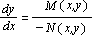The lecturer at this point will warn the students that they cannot possibly understand such higher mathematics, and will order them to take the method at face value, since "it works". Lecturers often raise their voices at this point, and students respond by turning to reading the school paper in class.
This fraudulent explanation demeans the students' intelligence while insinuating that the lecturer is in possession of some higher secret that the class is too stupid to share. Not exactly a celebration of the life of intellect.
Now let us see how integrating factors - and, incidentaliy, exact differential equations - can be explained simply and rigorously.
Step 1. Together with the differential equationone considers the plane autonomous system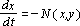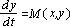It is of the utmost importance to explain the relation between the solutions of the differential equation and the solutions of the sytem. The solutions of the system are trajectories, they are parametric curves endowed with a velocity given by the vector field. The solutions of the corresponding differential equation are integral curves, and their graphs are the graphs of the trajectories deprived of velocity. Often, instead of solving the differential equation, it is more convenient to solve the corresponding autonomous system. Why? Because there are a great many piane autonomous systems that correspond to the same differential equation, namely all systems of the form
dxdt = -q(x,y)N(x,y),
dydt = q (x,y)M(x,y)

for any function q. For historical reasons, such systems are sometimes written in the quaint form

q Mdx + q Ndy = 0,

but one should bear in mind that this misleading notation is just another way of writing an autonomous system of differential equations.
Changing the factor q in a system changes the speed on the trajectories, while the integral curves remain the same. This phenomenon can and should be illustrated by striking geometric examples.
Step 2. After these preliminaries, the students are ready for the question: can we choose the factor q judiciousiy so as to be able to solve the system, and hence the differential equation? One may now appeal to the geometry of vector fields to motivate the choice of an integrating factor. The integrating factor is now introduced as the factor q that makes the vector field "best" in any one of several senses, both geometric and analytic, that the teacher may choose from. Exact differential equations are intuitively understood by the topographic interpretation of exact vector fields.
I hasten to add that I am not in the least "against" differentials. On the contrary I believe that very soon we will be forced to add an elementary course in the calculus of exterior differential forms to our undergraduate mathematics curriculum. At MIT we are already under pressure from some engineering departments to do so.
Lesson eight: Avoid word prohlems.
I once asked a colleague of mine why he so liked word problems, and his answer was: "I like them because one can assign good problem sets."
My colleague's answer betrayed a common error of reasoning. A striking instance of this error occurred in the old Cambridge Tripos before G.H. Hardy did away with it after a sarcastic critique. Students had to train for years for the tripos under the guidance of professional trainers. The best trainers were aware of all the tricks that could appear in a tripos problem, and would make sure that their students would employ the right tricks at the right time. The names of winners of the old Cambridge Tripos are now forgotten; very few mathematicians we have ever heard of ever won the Cambridge Tripos.
My colleague's error consists of believing that the more testable the material, the more teacheable it is. A wider spread of performance in the problem sets and in the quizzes makes the assignement of grades "more objective". The course is turned into a game of skill, where manipulative ability outweighs understanding.
The word problems that we find in differential equations textbooks are shameful. They are artificial, dishonest, unrealistic, contrived repetitive and irrelevant. I cannot see how a student can learn anything by heing forced to solve snowplow problems or Rube Goldberg flows of salt water in communicating tanks.
Most students take the differential equations course in order to master techniques to be later applied in solving the real word problems of their profession. The "word problems" a student of economics will meet are drastically different from the "word problems" of a student of chemical engineering. We cannot hope to encompass such a variety of "word problems" under the one umbrella of Mickey Mouse word problems.
Lesson nine: Motivate the Laplace transform.
Ordinarily, we motivate the Laplace transform by appealing to initial value problems for linear differential equations with constant coefficients. But this motivation is rather thin: taking inverse Laplace transforms is no joke and initial value problems can be solved in other ways.
I do not know how to properly motivate the Laplace transform; allow me to present some scattered comments.
1. Insofar as the Laplace transform goes, two radically different uses of the word "function" are dangerously confused with each other. The first is the ordinary notion of function as a something that has a graph. The second is the radically different notion of function as density, whether mass density or probability density. For the sake of the argument, let us agree to call this second kind of function "density function". Professional mathematicians have avoided facing up to density functions by a variety of escapes, such as Stieltjes integrals, measures, etc. But the fact is that the current notation for density functions in physics and engineering is provably superior, and we had better face up to it squarely.
2. Density functions are sometimes described by drawing their graphs, but this description is misleading. The "value" of a density function at a point is a meaningless term. What has meaning for a density function is the integral of a density function from a to b. Such an integral gives the mass contained in the interval [a, b], or the probability that a random variable takes values between a and b.
3. Once the idea of density functions is hammered in, it is easy to go to the next step, namely, to give a simple yet rigorous treatment of the Dirac delta function.
Indeed, since the value of a density function at a point is irrelevant, it follows that there is no reason whatsoever why a density function should have a graph at all. All a density function needs to have is an integral.
You have a problem if you believe that a "function" that has an integral should also have a graph. This prejudice should be gotten rid of as fast as possible. A unit mass at the point c is the simplest density function that does not have a graph. It is defined by stating that any integral of the Dirac delta function d c(x) over an interval [a, b] will equal zero if the interval does not contain the point c, and one if the interval contains the point c. From this definition, all properties of the Dirac delta function are easily derived without any hysterical appeals to functions taking infinite values. One should illustrate the method by computing the derivative of the Dirac delta function.
There is nothing wrong with keeping the functional notation for density functions - as physicists and engineers always did - as long as one bears in mind that density functions cannot be evaluated, but only integrated.
4. Whereas ordinary functions are multiplied in the usual way, it makes no physical sense to multiply density functions. Density functions have another kind of multiplication that makes sense, namely, convolution.
A good way to introduce students to convolution is to compute the convolution of two density functions each of which is the sum of Dirac delta functions: the convolution of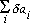with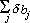is the density function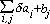Try it: you'll like it.
5. This item is more in the line of a personal confession.
Every time I teach the Laplace transform I feel a pang of remorse for something I think I ought to have done and I have not yet succeeded in doing. Without question, the most remarkable theorem about convolution and one of the least known, is the Titchmarsh convolution theorem. In its simplest form, it states that if the convolution of two functions is identically zero in the interval [O, b], then there exists an a [ b such that one of the functions is identically zero in the interval [0, a] and the other is identically zero in the interval 0, b - a].
No elementary proof of this theorem is known. Titchmarsh's proof uses high- powered complex variable methods. There is a phony-elementary proof due to Mikusinski. I would love to learn the "right" proof of the Titchmarsh convolution theorem before the end of my days.
Lesson ten: Teach concepts, not tricks.
What can we expect students to get out of an elementary course in differential equations? I reject the "bag of tricks" answer to this question. A course taught as a bag of tricks is devoid of educational value. One year later, the students will forget the tricks, most of which are useless anyway. The bag of tricks mentality is, in my opinion, a defeatist mentality, and the justifications I have heard of it, citing poor preparation of the students, their unwillingness to learn, and the possibility of assigning clever problem sets, are lazy ways out.
In an elementary course in differential equations, students should learn a few basic concepts that they will remember for the rest of their lives, such as the universal occurrence of the exponential function, stability, the relationship between trajectories and integrals of systems, phase plane analysis, the manipulation of the Laplace transform, perhaps even the fascinating relationship between partial fraction decompositions and convolutions via Laplace transforms. Who cares whether the students become skilled at working out tricky problems. What matters is their getting a feeling for the importance of the subject, their coming out of the course with the conviction of the inevitability of differential equations, and with enhanced faith in the power of mathematics. These objectives are better achieved by stretching the students' minds to the utmost limits of cultural breadth of which they are capable, and by pitching the material at a level that is just a little higher than they can reach.
We are kidding ourselves if we believe that the purpose of undergraduate teaching is the transmission of information. Information is an accidental feature of an elementary course in differential equations; such information can nowadays be gotten in much better ways than sitting in a classroom. A teacher of undergraduate courses belongs in a class with P.R. men, with entertainers, with propagandists, with preachers, with magicians, with gurus. Such a teacher will be successful if at the end of the course every one of his or her students feels they have taken "a good course", even though they may not quite be able to pin down anything specific they have learned in the course.